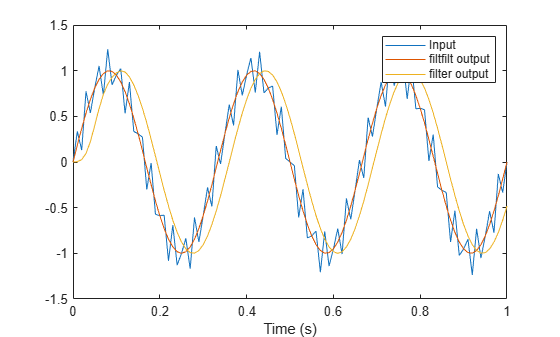# Anti-Causal, Zero-Phase Filter Implementation

In the case of FIR filters, it is possible to design linear phase filters that, when applied to data (using `filter` or `conv`), simply delay the output by a fixed number of samples. For IIR filters, however, the phase distortion is usually highly nonlinear. The `filtfilt` function uses the information in the signal at points before and after the current point, in essence "looking into the future," to eliminate phase distortion.

To see how `filtfilt` does this, recall that if the Z-transform of a real sequence $x\left(n\right)$ is $X\left(z\right)$, then the Z-transform of the time-reversed sequence $x\left(-n\right)$ is $X\left({z}^{-1}\right)$. Consider the following processing scheme:When $|z|=1$, that is $z={e}^{j\omega }$, the output reduces to $X\left({e}^{j\omega }\right){|H\left({e}^{j\omega }\right)|}^{2}$. Given all the samples of the sequence $x\left(n\right)$, a doubly filtered version of $x$ that has zero-phase distortion is possible.

For example, a 1-second duration signal sampled at 100 Hz, composed of two sinusoidal components at 3 Hz and 40 Hz, is

```fs = 100; t = 0:1/fs:1; x = sin(2*pi*t*3)+.25*sin(2*pi*t*40);```

Now create a 6th-order Butterworth lowpass filter to filter out the high-frequency sinusoid. Filter `x` using both `filter` and `filtfilt` for comparison:

```[b,a] = butter(6,20/(fs/2)); y = filtfilt(b,a,x); yy = filter(b,a,x); plot(t,x,t,y,t,yy) legend('Original','filtfilt','filter')```Both filtered versions eliminate the 40 Hz sinusoid evident in the original signal. The plot also shows how `filter` and `filtfilt` differ. The `filtfilt` line is in phase with the original 3 Hz sinusoid, while the `filter` line is delayed. The `filter` line shows a transient at early times. `filtfilt` reduces filter startup transients by carefully choosing initial conditions, and by prepending onto the input sequence a short, reflected piece of the input sequence.

For best results, make sure the sequence you are filtering has length at least three times the filter order and tapers to zero on both edges.